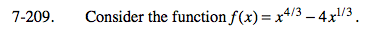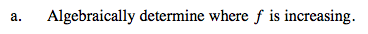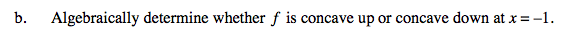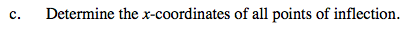### Home > CALC > Chapter Ch7 > Lesson 7.4.4 > Problem7-209

7-209.
1. Consider the function f(x) = x4/3 − 4x1/3. Homework Help ✎

1. Algebraically determine where f is increasing.

2. Algebraically determine whether f is concave up or concave down at x = −1.

3. Determine the x-coordinates of all points of inflection.Where is f '(x) positive?Solve f ''(x) > 0 and f ''(x) < 0.An inflection point occurs where f ''(x) undergoes a sign change.
Sometimes that happens where f ''(x) = 0 and sometimes where f ''(x) = DNE.